Courses

# Stress & Strain - MCQ Test 1

## 20 Questions MCQ Test RRB JE for Mechanical Engineering | Stress & Strain - MCQ Test 1

Description
This mock test of Stress & Strain - MCQ Test 1 for Mechanical Engineering helps you for every Mechanical Engineering entrance exam. This contains 20 Multiple Choice Questions for Mechanical Engineering Stress & Strain - MCQ Test 1 (mcq) to study with solutions a complete question bank. The solved questions answers in this Stress & Strain - MCQ Test 1 quiz give you a good mix of easy questions and tough questions. Mechanical Engineering students definitely take this Stress & Strain - MCQ Test 1 exercise for a better result in the exam. You can find other Stress & Strain - MCQ Test 1 extra questions, long questions & short questions for Mechanical Engineering on EduRev as well by searching above.
QUESTION: 1

### A 1.5 mm thick sheet is subject to unequal biaxial stretching and the true strains in the directions of stretching are 0.05 and 0.09. The final thickness of the sheet in mm is

Solution: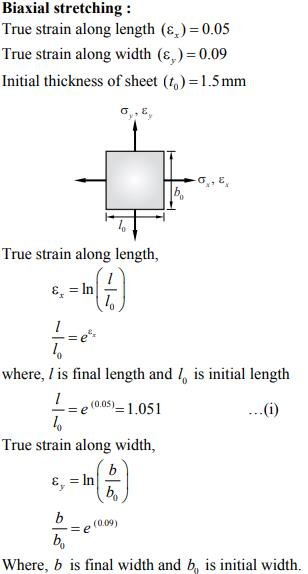QUESTION: 2

### A steel bar of 40 mm × 40 mm square cross-section is subjected to an axial compressive load of 200 kN. If the length of the bar is 2 m and E = 200 GPa, the elongation of the bar will be:

Solution: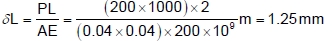QUESTION: 3

### The ultimate tensile strength of a material is 400 MPa and the elongation up to maximum load is 35%. If the material obeys power law of hardening, then the true stress-true strain relation (stress in MPa) in the plastic deformation range is:

Solution: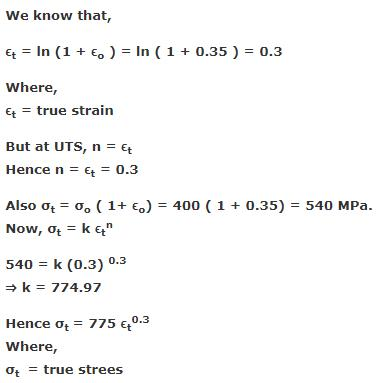QUESTION: 4

An axial residual compressive stress due to a manufacturing process is present on the outer surface of a rotating shaft subjected to bending. Under a given bending load, the fatigue life of the shaft in the presence of the residual compressive stress is:

Solution: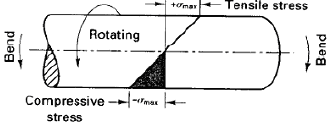A cantilever-loaded rotating beam, showing the normal distribution of surface stresses. (i.e., tension at the top and compression at the bottom)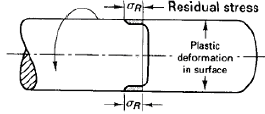The residual compressive stresses induced.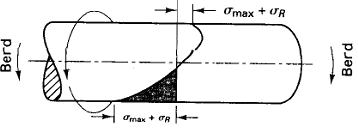Net stress pattern obtained when loading a surface treated beam. The reduced magnitude of the tensile stresses contributes to increased fatigue life.

QUESTION: 5

A static load is mounted at the centre of a shaft rotating at uniform angular velocity. This shaft will be designed for

Solution:

When shaft rotates at constant ω, each fibre of the shaft will undergo tensile and compressive loads. Therefore, the shaft is under the action of cyclic load.Hence the design should be for fatigue loading.

QUESTION: 6

Fatigue strength of a rod subjected to cyclic axial force is less than that of a rotating beam of the same dimensions subjected to steady lateral force because

Solution:

For fatigue loading normally generated tensile and compressive stresses in the shaft.

QUESTION: 7

A rod of length L and diameter D is subjected to a tensile load P. Which of the following is sufficient to calculate the resulting change in diameter?

Solution:

For longitudinal strain we need Young's modulus and for calculating transverse strain we need Poisson's ratio. We may calculate Poisson's ratio from E = 2G(1+μ) for that we need Shear modulus.

QUESTION: 8

A test specimen is stressed slightly beyond the yield point and then unloaded. Its yield strength will

Solution: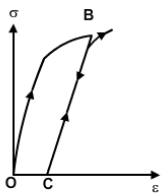QUESTION: 9

The deformation of a bar under its own weight as compared to that when subjected to a direct axial load equal to its own weight will be:

Solution:

Elongation of bar due to its own weight=Pl/2AE

Elongation of bar due to axial load=Pl/AE

Now comparing both..we will take the ratio.. (Pl/2AE)÷(Pl/AE)=1/2...i.e half

QUESTION: 10

Which one of the following statements is correct?

A beam is said to be of uniform strength, if

Solution:
QUESTION: 11

A bolt of uniform strength can be developed by

Solution:

A bolt of uniform strength can be developed by keeping the core diameter of threads equal to the diameter of the unthreaded portion of the bolt as Uniform strength means equal to threaded and unthreaded portion.

QUESTION: 12

A bar of length L tapers uniformly from diameter 1.1 D at one end to 0.9 D at the other end. The elongation due to axial pull is computed using mean diameter D. What is the approximate error in computed elongation?

Solution:

Actual elongation of the bar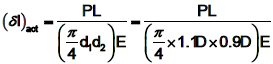Calculated elongation of the bar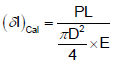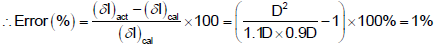QUESTION: 13

In the case of an engineering material under unidirectional stress in the x -direction, the Poisson's ratio is equal to (symbols have the usual meanings)

Solution:

Since stress is in x direction, longitudinal strain will develop in x direction. and lateral strain will be in y direction. Poisson's ratio= lateral strain/ longitudinal strain.

QUESTION: 14

If the value of Poisson's ratio is zero, then it means that

Solution:

Poisson's ratio is defined as lateral strain to longitudinal strain for value to be 0 lateral strain equals zero which means No change in diameter on application of axial load which is when the body doesn't deform i.e rigid body

QUESTION: 15

If a piece of material neither expands nor contracts in volume when subjected to stress, then the Poisson‟s ratio must be

Solution:

€v=0. (1-2¥)×(£x+£y+£z)/E =0. since body is constrained in all directions there will be equal stress in all directions. £x=£y=£z. 1-2¥=0. ¥=1/2= 0.5 ans.

QUESTION: 16

Which point on the stress strain curve occurs after proportionality limit?

Solution:

The curve will be stress-strain proportional upto the proportionality limit. After this, the elastic limit will occur.

QUESTION: 17

Assertion (A): Plastic deformation is a function of applied stress, temperature and strain rate.

Reason (R): Plastic deformation is accompanied by change in both the internal and external state of the material.

Solution:
• Following the elastic deformation, material undergoes plastic deformation.
• Also characterized by relation between stress and strain at constant strain rate and temperature.
• Microscopically…it involves breaking atomic bonds, moving atoms, then restoration of bonds.
• Stress-Strain relation here is complex because of atomic plane movement, dislocation movement, and the obstacles they encounter.
• Crystalline solids deform by processes – slip and twinning in particular directions.
• Amorphous solids deform by viscous flow mechanism without any directionality.
• Equations relating stress and strain are called constitutive equations.
• A true stress-strain curve is called flow curve as it gives the stress required to cause the material to flow plastically to certain strain.
QUESTION: 18

The correct sequence of creep deformation in a creep curve in order of their elongation is :

Solution:

Primary or transient creep occurs first. For secondary creep, sometimes termed steady-state creep, the rate is constant; that is, the plot becomes linear, finally tertiary creep occurs in which there is an acceleration of the rate and ultimate failure

QUESTION: 19

Consider the following statements:

For increasing the fatigue strength of welded joints it is necessary to employ

1. Grinding

2. Coating

3. Hammer peening

Of the above statements

Solution:

A polished surface by grinding can take more number of cycles than a part with rough surface.

In Hammer peening residual compressive stress lowers the peak tensile stress

QUESTION: 20

The number of elastic constants for a completely anisotropic elastic material which follows Hooke's law is:

Solution: